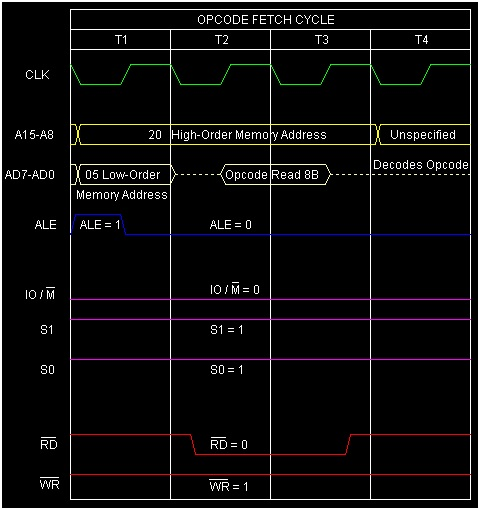# Instruction type ADC R in 8085 Microprocessor

In 8085 assembly language coding, sometimes there is a requirement to add two numbers and where each of these numbers are having several Bytes in size. As example, let us add the following two 16-bit numbers.

    1
10 50H
A0 F1H
------
B1 01H


In this example, the addition of 50H and F1H results in a sum of 01H with a carry of 1. Now, we are supposed to add 10H and A0H along with this carry of 1. To carry out such a calculation, 8085 supports us with suitable instructions to add two numbers along with carry value.

Here, ADC is a mnemonic that stands for ‘ADd with Carry’ and ‘R’ stands for any of the following registers, or memory location M pointed by HL pair.This instruction is mainly used to add contents of R register and Accumulator along with the carry value. Here the carry can be either 0 or 1. The result of this addition operation will be stored in the Accumulator itself overwriting its previous content. This is 1-Byte instruction so also occupies 1-Byte in the memory. So this R can have 8 possible values and thus 8 possible opcodes.

R = A, B, C, D, E, H, L, or M


Mnemonics, Operand Opcode(in HEX) Bytes

Let us consider ADC E as a sample instruction falling in this category. As mentioned, it is a 1-Byte instruction. Let us consider E register and Accumulator are initial having values 10H and A0H with initial Carry=1. Now if we execute the instruction ADC E, then the result B1H will remain stored on the Accumulator. The result of execution of this instruction is shown below with tracing table.

Before After

(E)

10H 10H

(A)

A0H B1H

(F)

Cy=1, Other flag bits may have any value Cy=0,AC=0,Z=0,P=1,S=1